Example Questions

2 Next →

Example Question #441 : Plane Geometry

A rhombus has two interior angles each with a measurement of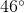. Find the measurement for one of the two remaining angles.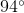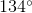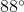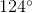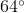Explanation:

First, consider that the sum total of the four interior angles in any rhombus must equal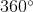. Secondly, a rhombus must have two sets of equivalent opposite interior angles, and a rhombus must have two sets of adjacent interior angles. The adjacent interior angles must be supplementary—meaning they have a sum total of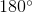One way to approach this problem is to realize that each of the remaining two angles must have the same measurement, and that each will be supplementary angles with. Therefore, find the difference betweenandto find the solution.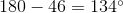Example Question #442 : Plane Geometry

A rhombus has two interior angles each with a measurement of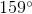. Find the measurement for one of the two remaining angles.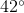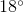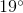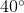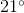Explanation:

A rhombus must have two sets of equivalent opposite interior angles, and a rhombus must have two sets of adjacent interior angles. The adjacent interior angles must be supplementary—meaning they have a sum total of.

One way to approach this problem is to realize that each of the remaining two angles must have the same measurement, and that each will be supplementary angles with. Find the difference betweenandto find the solution.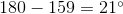Example Question #443 : Plane Geometry

A rhombus has two interior angles each with a measurement of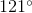. Find the sum of the remaining two angles.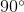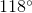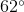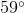Explanation:

To solve this problem, consider that the sum total of the four interior angles in any rhombus must equal. Furthermore, a rhombus must have two sets of equivalent opposite interior angles, and a rhombus must have two sets of adjacent interior angles. The adjacent interior angles must be supplementary—meaning they have a sum total of.

Since this problem provides the measurement for two of the interior angles, find the sum of those two angles. Then subtract that sum fromto find the sum of the two remaining interior angles.

The solution is: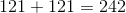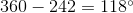Note: this means that each of the two remaining angles must have a measurement of.

2 Next →